# 2nd PUC Physics Question Bank Chapter 1 Electric Charges and Fields

## Karnataka 2nd PUC Physics Question Bank Chapter 1 Electric Charges and Fields

### 2nd PUC Physics Electric Charges and Fields NCERT Text Book Questions and Answers

Question 1.
What is the force between two small charged spheres having charges of 2 × 10-7 and 3 x 10-7 C placed 30cm apart in the air?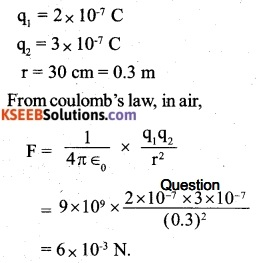Question 2.
The electrostatic force on a small sphere of charge 0.4 µ C due to another small sphere of charge – 0.8 µ C in air is 0.2 N.
(a) What is the distance between the two spheres?
(b) What is the force on the second sphere due to the first?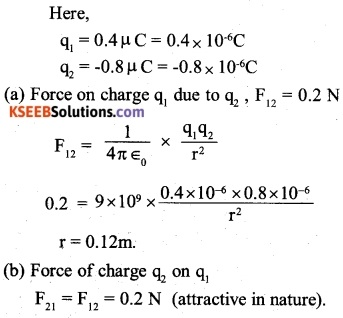Question 3.
Check that the ratio ke2/Gme mp is dimensionless. Lookup a table of Physical constants and determine the value of this ratio. What does the ratio signify?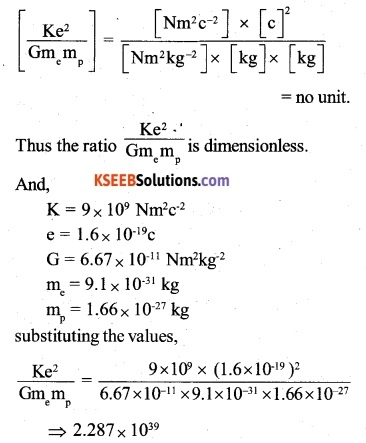This value represents the ratio of the electrostatic force of gravitational force between an electron and a proton.

Question 4.
(a) Explain the meaning of the statement ‘electric charge of a body is quantized.
(b) Why can one ignore quantization of electric charges when dealing with macroscopic i.e large-scale charges?
(a) Total charge on a body is equal to integral multiple of charge on an electron (e).
i.e. q = ± ne, where n = 0, 1, 2, 3, ….
(b) At macroscopic level, the quantisation of charge has no practical importance because the charge at macroscopic level is very large as compared to elementary charge i.e. 1.6 x 10_19C. For example, a small charge of 1 μC  has about 1013 electronic charges. In such cases the charge may be treated as continuous and not quantised.Question 5.
When a glass rod is rubbed with a silk cloth, charges appear on both. A similar phenomenon is observed with many other pairs of bodies. Explain how this observation is consistent with the law of conservation of charge.
On rubbing, the electrons are transferred from one body to the other. For example, when a glass rod is rubbed with a piece of silk cloth, electrons from the glass rod are transferred to the piece of silk cloth. The glass rod acquires (+) charge whereas the silk cloth gets a (-) charge. Silk and glass are neutral before rubbing and after rubbing the total charges on the glass as well as silk taken together are once again zero. A similar phenomenon is observed with other pairs of bodies. Thus, in an isolated system, the total charge is neither created nor destroyed, the charge is simply transferred from one body to the other. This observation is consistent with the law of conservation of charges.

Question 6.
Four-point charges qA = 2 µC, qB = -5 µC, qc = 2 µC and qD = -5 µC are located at the corners of a square ABCD of side 10cm. What is the force of a charge of 1 µC placed at the centre of the square?
Consider the square ABCD of each side 10cm and centre O. The charge of 1 µC is placed at point O. The charge of 1 µC is placed at point O.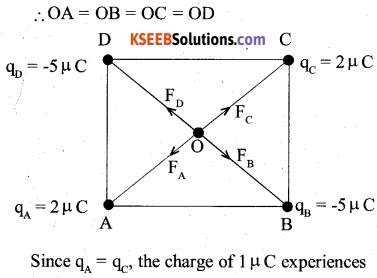equal and opposite forces due to the charges qA and qc. Similarly, the charges of 1 µC experiences equal and opposite forces Ffi and Fd due to the charges qB and qD.

Hence, the net force on the charge of 1 pC due to the given arrangement of the charges is zero.

Question 7.
(a) An electrostatic field line is a continuous curve. That is a Held line cannot have sudden breaks. Why not?
(b) Explain why two field lines never cross each other at any point?
(a) An electrostatic field line starts from a positive charge and ends at a negative charge. The tangent to this line at any point represents the direction of the field at that point. Since electric field is continuous so the field line has to be continuous.

(b) If two field lines cross each other, then at the point of intersection, there will be two tangents showing that at the point of intersection, there are two directions of a given electric field. This is not possible. Hence, two field lines cannot cross each other.

Question 8.
Two point charges qA = 3 µC and qB = -3 µC are located 20 cm apart in vacuum.
(a) What is the electric field at the midpoint O of the line AB joining the two charges?
(b) If a negative test charge of magnitude 1.5×10-9C is placed at this point, what is the force experienced by the test charge?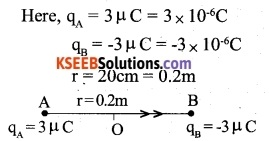The force on the negative charge acts in a direction opposite to that of the electric field.Question 9
A system has two charges qA = 2.5× 10-7C and qB = -2.5 x 10-7C located at points
A: (0, 0, -15cm), respectively. What are the total charge and electric dipole moment of the system?
The charges qA and qB are located at the points A (0,0, – 15cm) and B (0, 0, +15cm). B The points A and B lie on z-axis as shown.
It follows that,
AB = OA + OB = 30ci = 0.3m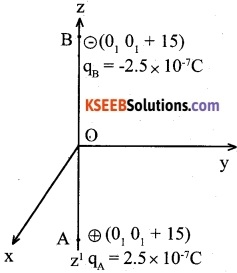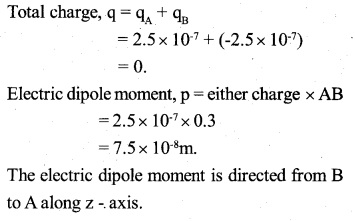Question 10.
An electric dipole with dipole moment 4×10-9cm is aligned at 30° with the direction of a uniform electric field of magnitude 5 × 104 NC1. Calculate the magnitude of the torque acting on the dipole.Question 11.
A polythene piece rubbed with wool is found to have a negative charge of 3x 10 7C
(a) Estimate the number of electrons transferred (from which to which ?)
(b) Is there a transfer of mass from wool to polythene?
(a) The total charge transferred,
q = -3 x 10-7C
charge on an electron, e = -1.6x 1019C From quantization of charge, q = ne Therefore, number of electrons transferred,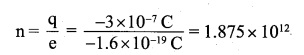(b) Since electrons are transferred from wool to polythene, there is transfer of mass also. This is because electrons are material particles and possess mass (me = 9.1 x 10-31kg).

Question 12
(a) Two insulated charged copper spheres A and B have their centres separated by a distance of 50 cm. What is the mutual force of electrostatic repulsion if the charge on each is 6.5 x 10-7C? The radii of A and B are negligible compared to the distance of separation.
(b) What is the force of repulsion if each sphere is charged double the above amount and the distance between them is halved?
(a) Charge on sphere A, q1, = 6.5 x 10-7c
charge on sphere B, q2 = 6.5 x 10 7c
Distance between the spheres A and B, r = 50 cm= 0.5mQuestion 13
Suppose the spheres A and B in exercise 1.12 have identical sizes. A third sphere of the same size but uncharged is brought in contact with the first, then brought in contact with the second, and finally removed from both. What is the new force of repulsion between A and B?
The charge on A and B, q1 = q2 = 6.5x 107C.
The distance between the spheres r = 0.5m. Since the spheres are the same size, they will possess equal charges on being brought in contact. When uncharged sphere c (q3 = 0) is bought in contact with A, charge left on A.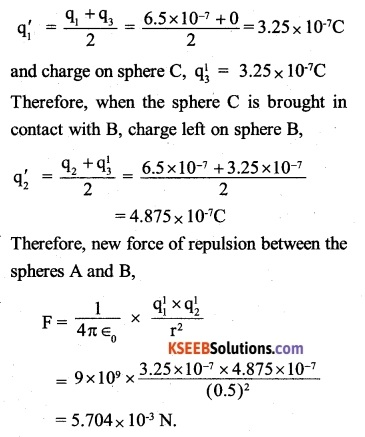Question 14.
Fig 1.33 shows tracks of three charged particles in a uniform electrostatic field. Give the signs of the three charges. Which particle has the highest charge to mass ratio?Charges (1) and (2) are negative as they were attracted by the positive plate and charge (3) is positive as it is deflected towards negative plate.
Thus charge (3) has highest charge to mass ratio (as it would be a positive figure)

Question 15.
Consider a uniform electric Held $$\vec { E } =3\times { 10 }^{ 3 }\hat { i } { N }/{ C }$$
(a) What is the flux of this field through a spheres A and B, charges inside the box. Therefore, if the net outward flux is zero, we cannot conclude that the charge inside the box is zero. One can only say that the net charge inside the box is zero.
(b) A point charge +10 p C is a distance 5cm directly above the centre of a square of side 10cm, as shown in fig. 1.34. What is the magnitude of the electric flux through the square of 10cm on a side whose plane is parallel to the yz plane?
(b) What is the flux through the same square if the normal to its plane makes a 60° angle with the x-axis ?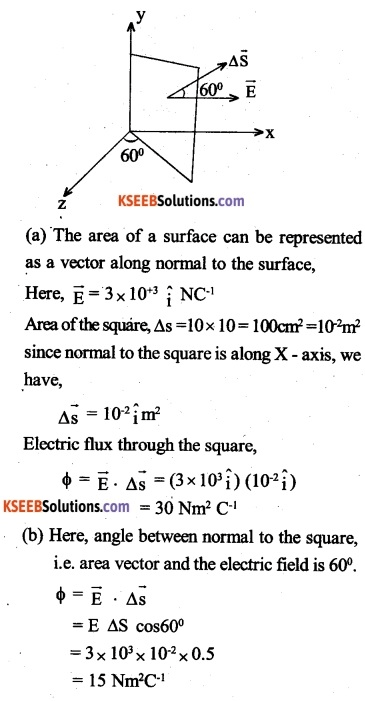Question 16.
What is the net flux of the uniform electric field of exercise 1.15 through a cube of side 20cm oriented so that its faces are parallel to the coordinate planes?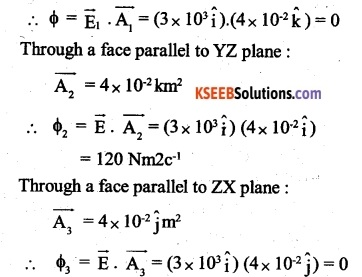In each plane, there is a set two faces of the cube. Through one face, electric flux enters and through the other face, an equal flux leaves. Therefore, net flux through the cube,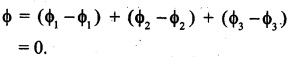Question 17.
Carefid measurement of the electric field at the surface of a black box indicates that the net outward flux through the surface of the box is 8.0 ×103Nm2/C.
(a) What is the net charge inside the box?
(b) If the net outward flux through the surface of the box were zero, could you conclude that there were no charges inside the box? Why or why not?(b) No, only that the net charge inside is zero.Question 18.
A point charge +10 µ C is a distance 5cm directly above the centre of a square of side 10cm, as shown in fig. 1.34. What is the magnitude of the electric flux through the square?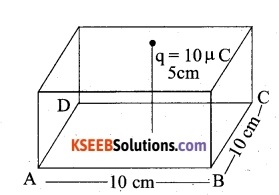consider that the charge q is at a distance of 5 cm from the square ABCD of each side 10 cm as shown. The square ABCD can be considered as one of the six faces of a cube of each side 10cm. Then according to Gauss’ theorem, total electric flux through all the six faces of the cube,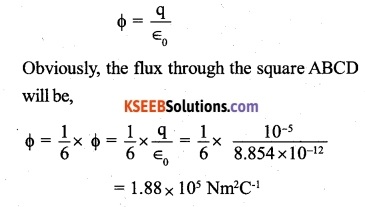Question 19.
A point charge of 2 p C is at the centre of a cubic Gaussian surface 9cm on edge. What is the net electric flux through the surface?
Here, q = 2µC = 2 × 10-6C
side of cube, a = 9cm = 0.09 m
According to Gauss’ law, the net electric flux through all the six faces of the cube,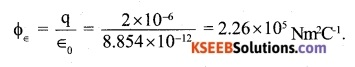Question 20.
A point charge causes an electric flux of  1 x 103Nm2/C to pass through a spherical Gaussian surface of a 10cm radius centred on the charge.
(a) If the radius of the Gaussian surface were doubted, how much flux would pass through the surface?
(b) What is the value of the point charge?
Here φ = -1 x 103Nm2C-1
radius of Gaussian surface, r = 10cm = 0. 1m.
(a) Flux does not change since the total charge q enclosed by the surface does not change.

(b) Let q be the charge inside the surface: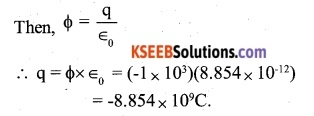Question 21.
A conducting sphere of radius 10cm has an unknown charge. If the electric field 20cm from the centre of the sphere is 1.5 x 103N/C and the points radially inward, what is the net change on the sphere?
R = (=10cm = 0.1 m) and centre O.
Let the charge on the sphere be ‘q’.
Further, let ‘P’ be a point at a distance r = 20cm from the centre of the sphere.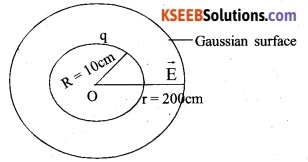The electric field E( = 1.5103NC-1) is directed radially inward as shown. So as to enclose the charged sphere, draw a sphere (gaussian surface) with the point O as centre and r as the radius. As the electric field is radial, it is normal to the gaussian surface everywhere. Therefore, electric flux through the gaussian surface,Question 22.
A uniformly charged conducting sphere of 2.4 m diameter has a surface charged density of 80 µ c/m2
(a) Find the charge on the sphere.
(b) What is the total electric flux leaving the surface of the sphere?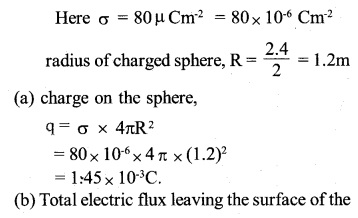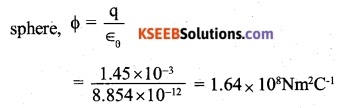Question 23.
An infinite line charge produces a field of 9 x 104N/C at a distance of 2cm. Calculate the linear charge density.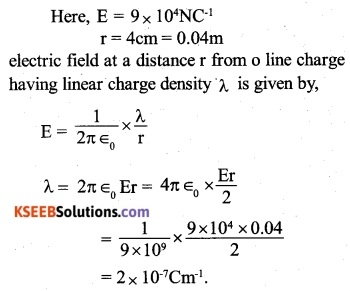Question 24.
Two large thin metal plates are parallel and close to each other. On their inner faces, the plates have surface charge densities of opposite signs and of magnitude 17x 10-22C/m2. What is $$\vec { E }$$:
(a) In the outer region of the first plate
(b) In the outer region of the second plate, and
(c) Between the plates?
Here, σ= 17 x 10-22 Cm-2
(a) To the left of plates.
The electric field at this region is zero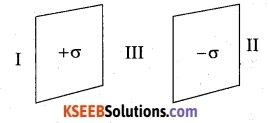(b) To the right of the plates
The electric field in this region is zero.

(c) Between the two plates
The electric field in this region is given by### 2nd PUC Physics Electric Charges and Fields Additional Exercises

Question 25.
An oil drop of 12 excess electrons is hold stationery under a constant electric field of 2.55 x 104 NC-1 in is 1.26 g em-3. Estimate the radius of the drop, (g = 9.81 ms-2; e = 1.60×10 19c)Question 26.
Which among the curves shown in Fig 1.35 cannot possibly represent electrostatic field lines?(a) It is not. Because field lines are not normal to the surfaces of the conductors.
(b) It is not, because, field lines cannot start from a negative charge.
(c) It is a correct representation of electric field lines.
(d) It is not because field lines cannot intersect each other.
(q) It is not, because field lines cannot form closed loops without having touching the source or any charge.Question 27.
In a certain region of space, the electric field is along the z-direction throughout. The magnitude of the electric field is, however, not constant but increases uniformly along the positive z-direction, at the rate of 105Nc1 per meter. What are the force and torque experienced by a system having a total dipole moment equal to 10-7cm in the negative z-direction?
Suppose that a dipole consisting of charges – q at A and +q at B is placed along the z-axis, such that it has dipole moment along the negative z-direction. The dipole moment of the dipole along the z-axis, PZ= -10-7Cm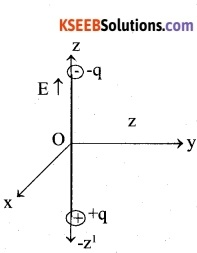The negative sign has been taken for the reason that the dipole moment is along the x negative z-axis.
The electric field is applied along the positive direction of the z-axis, such that (along the z-axis) In a non – uniform electric field, the force on the dipole is given by,The negative sign shows that force on the dipole is along the negative z-axis.
Since the electric dipole moment is along the negative z-axis and the electric field is along the positive z-axis, θ = 180°.
Hence, torque on the dipole, τ = pE sin 180° = 0.

Question 28.
(a) A conductor A with a cavity as shown in Fig. 1.36 (a) is given a charge Q. Show that the entire charge must appear on the outer surface of the conductor.(b) Another conductor B with charge q is inserted into the cavity keeping B insulated from A. Show that the total charge on the outside surface of A is Q + q [Fig. 1.36(b)].
(c) A sensitive instrument is to be shielded from the strong electrostatic fields in its environment. Suggest a possible way.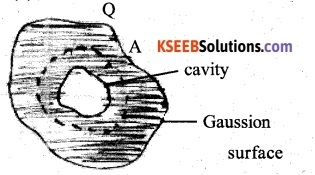Consider the gaussian surface (dotted) so as to enclose the cavity by lying wholly inside the conductor A. Since the electric field inside a conductor is zero, the part of the conductor within the cavity does not contain any net charge. By extending the same argument, no charge can be present inside the Gaussian surface, which lies just within the conductor. Hence the entire charge A must appear on the outer surface of conductor A.

consider the Gaussian surface (dotted), it encloses the cavity and the conductor B with charge q inserted in the cavity.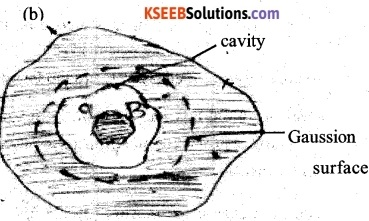As such, the electric flux will cross the gaussian surface and it would imply that the electric field exists inside conductor A. But the electric field inside conductor A must be zero. It will be true, only if the charge q on conductor B induces charge-q on the inner surface and +q on the outer surface of the

Conductor A. As there is already a charge +Q on the outer surface of the conductor A, the total charge on its outer surface becomes Q+q.

(c) The electric field inside a metallic conductor is zero. Therefore, instrument can be shielded from the strong electrostatic fields by enclosing n it with a hollow metallic structure.

Question 29.
A hollow charged conductor has a tiny hole cut into its surfaces. Show that the electric field in the hole is $$\left( { \sigma }/{ { 2\epsilon }_{ 0 } } \right) \hat { i }$$, where q is the unit vector in the outward normal direction and a is the surface charge density near the hole.
Let $$\vec { E }$$be the electric field in the hole due to rest of the charged conductor. In order to find $$\vec { E }$$ consider that the hole in the conductor is plugged. Let $$\vec { E }$$ be the electric field due to the charged conductor at a point’ outside it then,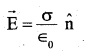After plugging the hole, the conductor becomes a closed one, the electric field inside it must be zero. It implies that inside the conductor, electric field due to the rest of the conductor must be equal and opposite to that due to the small portion of the conductor, that plugs the hole. However, outside the conductor, the two fields are in the same direction i.e. along the outward normal to the surface of the conductor. It, therefore, follows that the electric field in the hole due to the rest of the conductor,Question 30.
Obtain the formula for the electric field due to a long thin wire of uniform linear charge density X, without using Gauss’ law
Consider a long thin wire of uniform linear charge density λ  Let us find electric field due to the wire at the point P at a normal distance. PN = r from the wire Let AB = dx be a small elementary portion of the wire at a distance x from point N.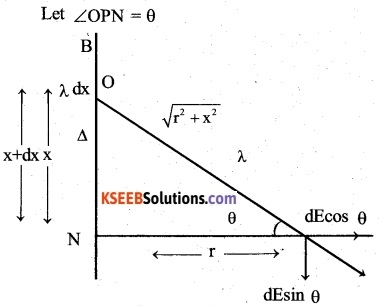Then charge on the elementary portion AB, dq= λ dn

Therefore, electric field due to the small portion AB at the point P,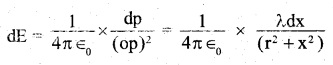The component dE sin θdue to the different small portions, such as AB, will cancel each other. Therefore, effective electric field due to the small portion AB,Question 31.
It is now believed that protons and neutrons are themselves built out of more elementary of charge $$+\frac { 2e }{ 3 }$$ and then’ down quarks’ denoted by ‘d’ of charge $$-\frac { e }{ 3 }$$ together with electrons build up ordinary matter. Suggest a possible quark composition of a proton and neutron.
The charge on up quark (u) = $$+\frac { 2e }{ 3 }$$
The charge on down quark = $$-\frac { e }{ 3 }$$
The charge on a proton is +e and it is made of three quarks. Therefore the possible quark composition of a proton is ‘uud

On the other hand, the neutron is a neutral particle but it is also made of three quarks. For this, the possible composition of the neutron is ‘udd’.

Question 32.
(a) Consider an arbitrary electrostatic field configuration. A small test charge is placed at a null point of the configuration. Show that the equilibrium of the test charge is necessarily unstable.
(b) Verify the result for the simple configuration of two charges of the same magnitude and sign placed a certain distance apart.
(a) Let the given system be in stable equilibrium. To check the stability the test charge is displaced slightly. For a stable equilibrium, the test charge should experience a restoring force pulling it towards the mean position. In other words, there are inward lines of force near the mean position or null point. But as per Gauss’s law, a closed surface enclosing no charge cannot have electric lines of force or flux. Thus, the equilibrium cannot be stable.
(b) Here the said midpoint is infected a null point. If the test charge is displaced axially i. e. along the line joining the two charges, a restoring force acts on the test charge. But if the test charge is slightly displaced normal to the line, the net force makes the charge move away from the null point. Thus, the test charge is not enjoying stable equilibrium.Question 33.
A particle of mass m and charge -q enters the region between the two plates initially moving along X-axis with speed $${ V }_{ \lambda }$$. The length The length of plate is L and a uniform electric field £ is maintained between the plates. Show that the vertical deflection of the particle at the far edge of the plate is $$-\frac { qE{ L }^{ 2 } }{ 2m{ v }_{ \lambda }^{ 2 } }$$
Let y be the vertical deflection of the charged particle since the particle is initially moving along x-axis, its initial speed along the vertical.
The electric field E is directed along vertical. Therefore, acceleration of the particle along the vertical, $${ U }_{ y }=0$$
The time for which the force due to the electric field acts on the particle,
$${ a }_{ y }=\frac { qE }{ m }$$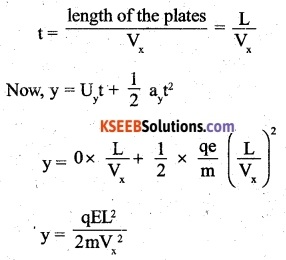Question 34.
Suppose that the particle in exercise 1.33 is an electron projected with velocity Vx = 2x 106ms1. If E between the plates separated by 0.5 cm is 9.1 x 102Nc_1, where will the electron strike the upper plate? Given, e = 1.6 x 1019C; and Me = 1 x 1031 kg.
Suppose that the electron strikes the upper plate at a distance x from the end, where it enters the electric field. Therefore the expression for vertical deflection becomes### 2nd PUC Physics Electric Charges and Fields Additional Entrance Examination Questions and Answers

Question 1.
A charge Q is placed at each of the opposite corners of a square. A charge q is placed at each
of the other two corners. If the net electrical force on the Q is zero, then ~ equals
(A) -1
(B) 1
(C) $$-2\sqrt { 2 }$$
(D) $${ -1 }/{ \sqrt { 2 } }$$
(C) $$-2\sqrt { 2 }$$

Question 2.
Four charge equals to -Q are placed at the four corners of a square and a charge q is at its centre. If the system is in equilibrium, the value of q is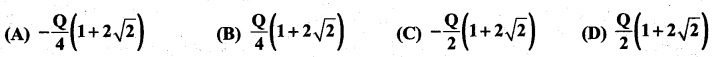(A)

Question 3.
If the amounts of electric flux entering and leaving an enclosed surface respectively are $${ \phi }_{ 1 }$$ and <$${ \phi }_{ 1 }$$ the electric charge inside the surface will be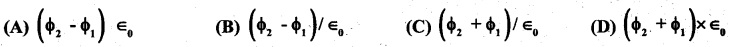(A)

Question 4.
A charged particle q is placed at the centre O of the cube (ABCDEFGH) of length L. Another same charge q is placed at a distance L from 0. Then, the electric flux through ABCD is.(C)

Question 5.
A thin spherical shell of radius R has charge Q spread uniformly over its surface. Which of the following graphs most closely represents the electric field E (r) produced by the shell in the range o≤ r < ∞ where r is the distance from the centre of the shell ?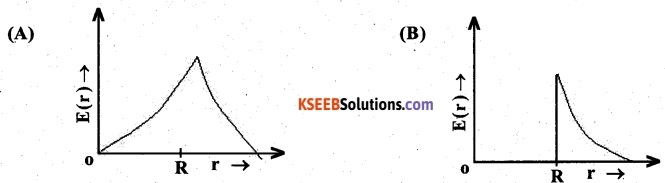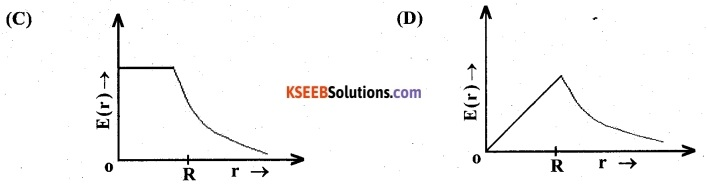(B)

Question 6.
Two-point charge +8q and -2q are located at x = 0 and x = L respectively. The location of a point on z-axis at which the net electric field due to these two point charges is zero is (CET 2009)
(A) 2L
(B) L/4
(C) 8L
(D) 4L